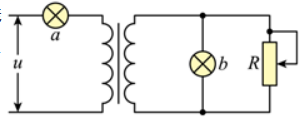$\text{A.}$ 灯泡的额定电压是 $44 \mathrm{~V}$ $\text{B.}$ 副线圈中交变电流的频率为 $12.5 \mathrm{~Hz}$ $\text{C.}$ 当滑动变阻器的滑片向下滑动时, 灯泡 $a$ 变暗 $\text{D.}$ 当滑动变阻器的滑片向下滑动时, 变压器输入功率变小

A

#### 解析：

$B$ 、由 $u=220 \sqrt{2} \sin 10 \pi t(V)$ 知 $\omega=10 \pi \mathrm{rad} / \mathrm{s}$, 则交变电流的频率为 $f=\frac{\omega}{2 \pi}=\frac{10 \pi}{2 \pi} H z=5 H z$, 理想变压器不改变交流电的 频率, 因此副线圈中交变电流的频率为 $5 \mathrm{~Hz}$,故 $B$ 错误；
C、当滑动变阻器的滑片向下滑时, 滑动变阻器的有效阻值减小, 副线圈电流变大, 根据 $\frac{I_1}{I_2}=\frac{n_2}{n_1}$ 可知原线圈电流变大, 流 过 $a$ 的电流增大, 灯泡 $a$ 变亮, 故 $C$ 错误；
$D$ 、当滑动变阻器的滑片向下滑动时, 根据 $C$ 选项的分析可知原线圈电流变大, 根据 $P=U I_1$ 可知电路总功率变大, 灯泡 $a$ 的功率也变大, 变压器输入功率不一定变小, 故 $D$ 错误。